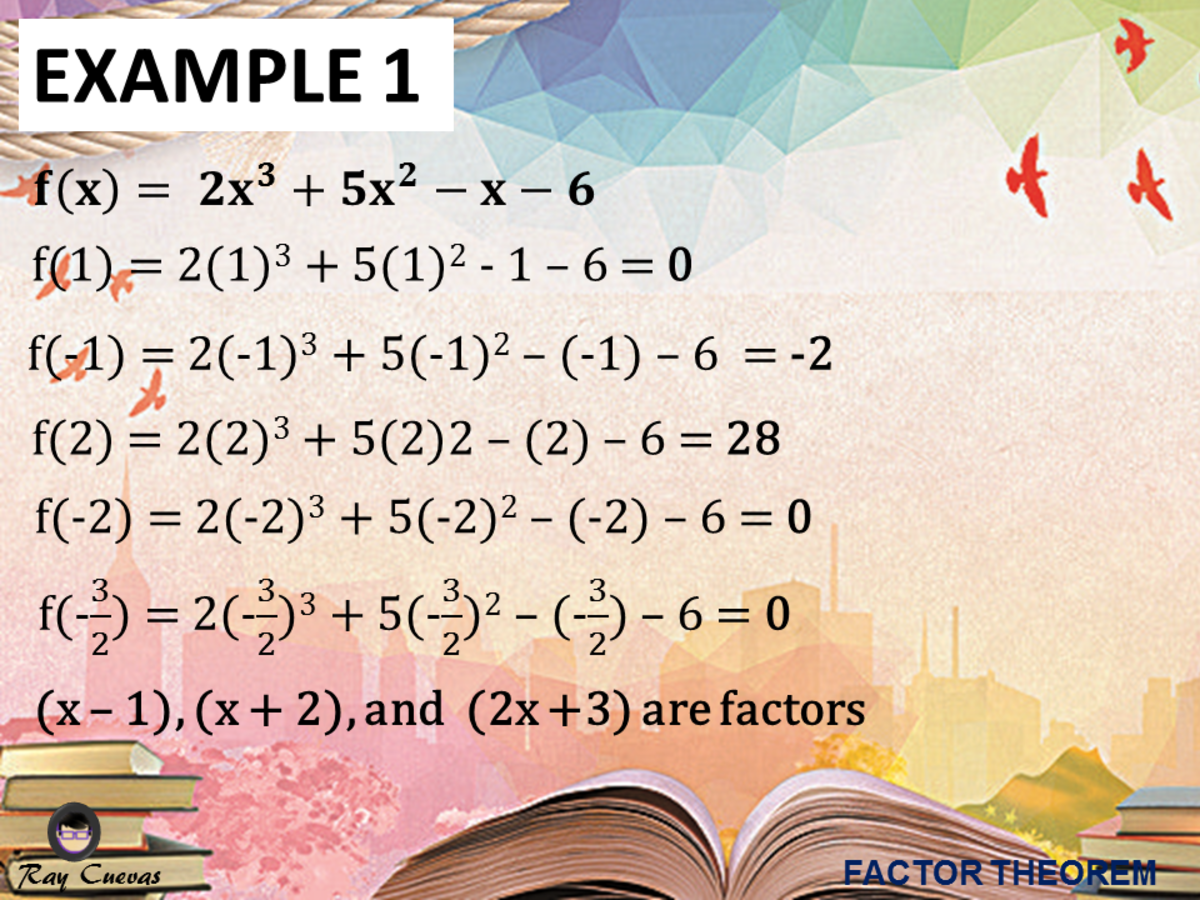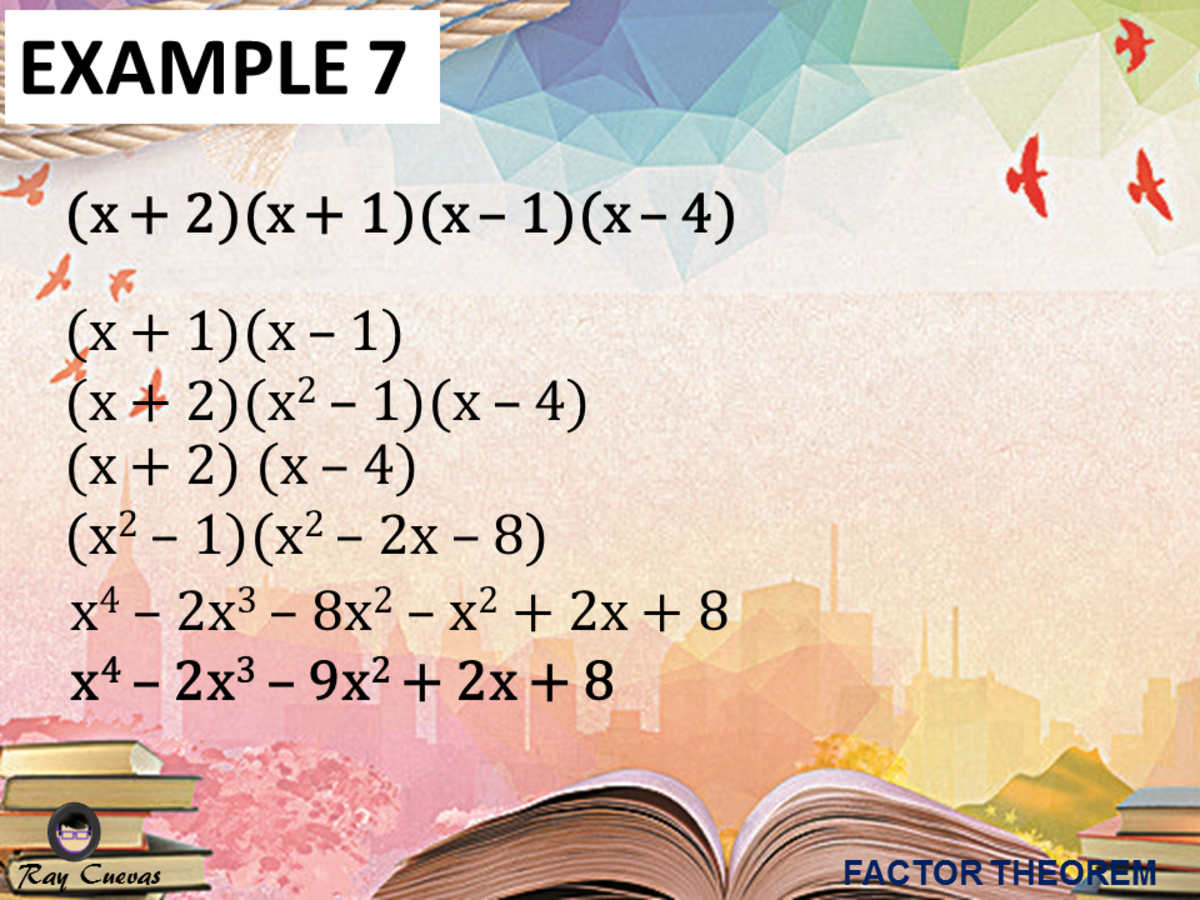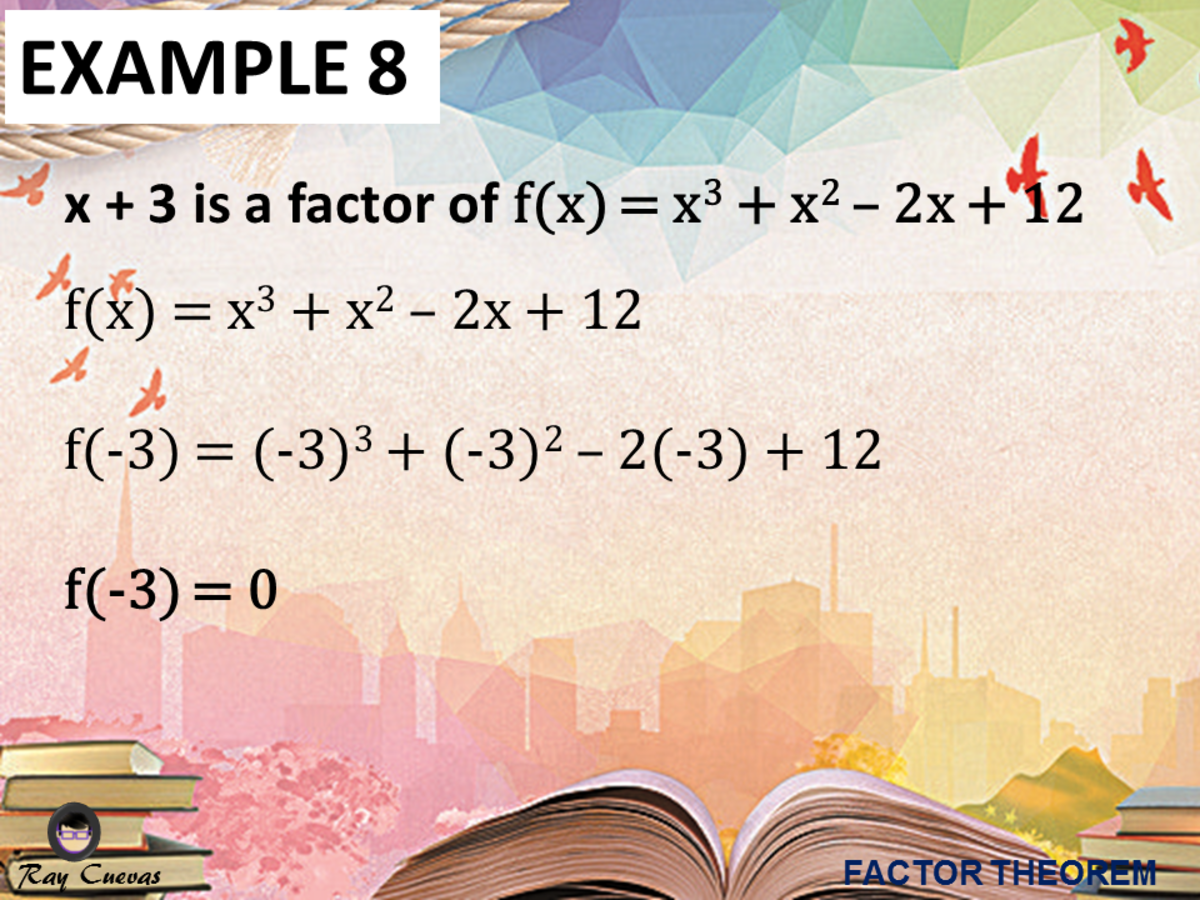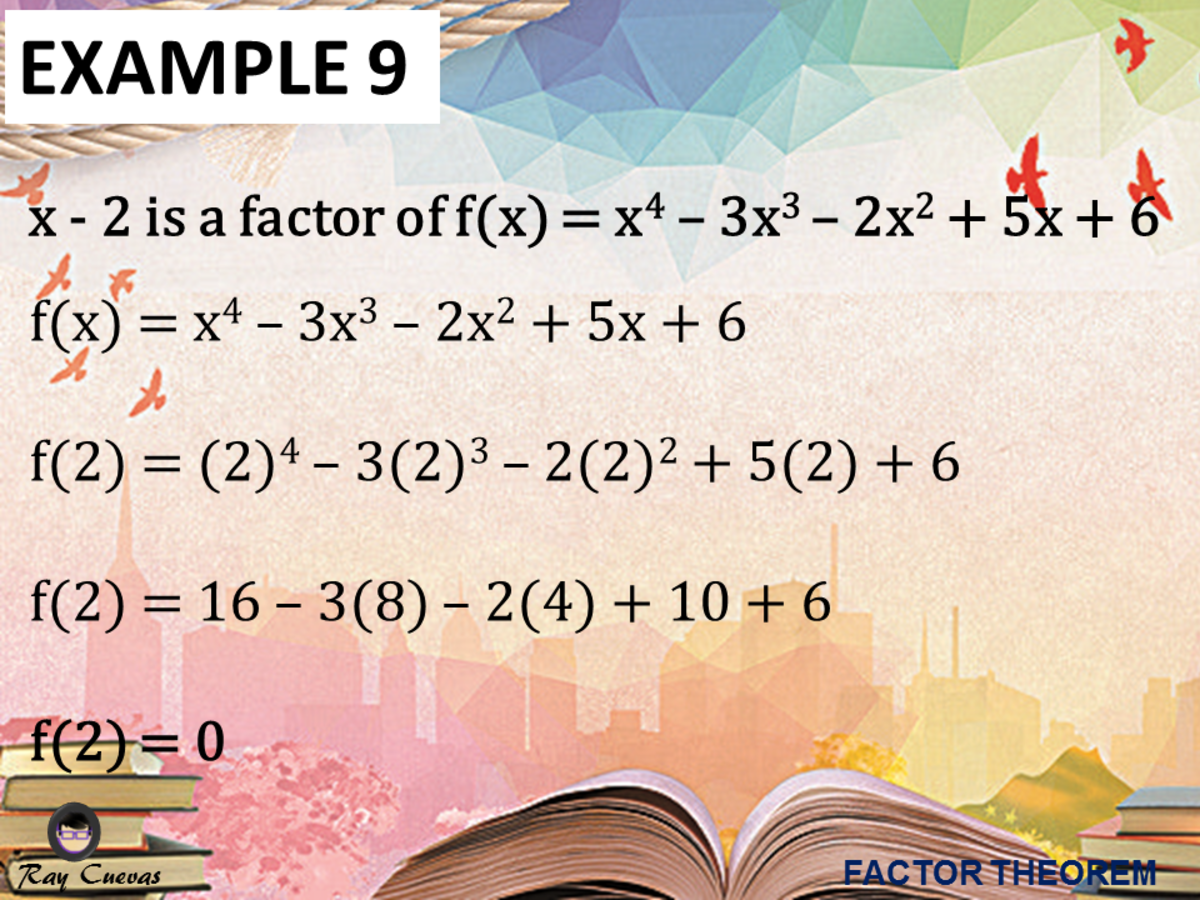Updated date:

# Using the Factor Theorem in Finding the Factors of Polynomials (With Examples)

Author:

Ray is a Licensed Engineer in the Philippines. He loves to write any topic about mathematics and civil engineering.

## What Is the Factor Theorem?

Factor Theorem is a particular case of the Remainder Theorem that states that if f(x) = 0 in this case, then the binomial (x – c) is a factor of polynomial f(x). It is a theorem linking factors and zeros of a polynomial equation.

Factor Theorem is a method that allows the factoring of polynomials of higher degrees. Consider a function f(x). If f(1) = 0, then (x-1) is a factor of f(x). If f(-3) = 0 then (x + 3) is a factor of f(x). The factor theorem can produce the factors of an expression in a trial and error manner. The Factor Theorem is useful for finding factors of polynomials.

There are two ways to interpret the Factor Theorem's definition, but both imply the same meaning.

Definition 1

A polynomial f(x) has a factor x – c if and only if f(c) = 0.

Definition 2

If (x – c) is a factor of P(x), then c is a root of the equation P(x) = 0, and conversely.

## Factor Theorem Proof

If (x – c) is a factor of P(x), then the remainder R obtained by dividing f(x) by (x – r) will be 0.

P(x) = (x – c) x Q(x)

Divide both sides by (x – c). Since the remainder is zero, then P(r) = 0.

P(x) / (x – c) = Q(x) + [0 / (x – c)

Conversely, if c is a root of P(x) = 0, P(c) = 0, then R = 0. Then,

P(x) / (x – c) = Q(x) + [0 / (x – c)]

P(x) = (x – c) x Q(x) + 0

P(x) = (x – c) x Q(x)

Therefore, (x – c) is a factor of P(x).

## Example 1: Factorizing a Polynomial by Applying the Factor Theorem

Factorize 2x3 + 5x2 – x – 6.

Solution

Substitute any value to the given function. Say, substitute 1, -1, 2, -2, and -3/2.

f(1) = 2(1)3 + 5(1)2 - 1 – 6

f(1) = 0

f(-1) = 2(-1)3 + 5(-1)2 – (-1) - 6

f(-1) = -2

f(2) = 2(2)3 + 5(2)2 – (2) - 6

f(2) = 28

f(-2) = 2(-2)3 + 5(-2)2 – (-2) - 6

f(-2) = 0

f(-3/2) = 2(-3/2)3 + 5(-3/2)2 – (-3/2) - 6

f(-3/2) = 0

The function resulted to zero for values 1, -2, and -3/2. Hence using the Factor Theorem, (x – 1), (x + 2), and 2x +3 are factors of the given polynomial equation.

(x – 1), (x + 2), (2x + 3)Example 1: Factorizing a Polynomial by Applying the Factor TheoremJohn Ray Cuevas

## Example 2: Using the Factor Theorem

Using the Factor Theorem, show that x – 2 is a factor of f(x) = x3 – 4x2 + 3x + 2.

Solution

We need to show that x – 2 is a factor of the given cubic equation. Start by identifying the value of c. From the given problem, the variable c is equal to 2. Substitute the value of c to the given polynomial equation.

f(x) = x3 – 4x2 + 3x + 2

f(2) = (2)3 – 4(2)2 + 3(2) + 2

f(2) = 8 – 16 + 6 + 2

f(2) = 0

Since f(2) = 0, we see from the factor theorem that x – 2 is a factor of f(x). Another method would be to divide f(x) by x – 2 and show that the remainder is zero. The quotient in the division would be another factor of f(x).

## Example 3: Finding a Polynomial with Prescribed Zeros

Find a polynomial f(x) of degree 3 with zeros 2, -1, and 3.

Solution

By the factor theorem, f(x) has factors x – 2, x + 1, x -3.

f(x) = a (x – 2)(x + 1)(x – 3)

Thus, where any nonzero value may be assigned to a. If we let a = 1 and multiply to the factors, we obtain a polynomial of degree three. Same goes as you use different values of a since it will still be reduced to lowest and most simple equation.

f(x) = (x – 2)(x + 1)(x – 3)

f(x) = (x2 + x – 2x – 2)(x – 3)

f(x) = x3 + x2 – 2x2 – 2x – 3x2 – 3x + 6x + 6

f(x) = x3 – 4x2 + x + 6

The polynomial of degree 3 that has zeros 2, -1, and 3 is x3 – 4x2 + x + 6.

## Example 4: Proving an Equation Is a Factor of a Quadratic Equation

Show that (x + 2) is a factor of P(x) = x2 + 5x + 6 using the Factor Theorem.

Solution

Substitute the value of c = -2 to the given quadratic equation. Prove that x + 2 is a factor of x2 + 5x + 6 using the Factor Theorem.

P(-2) = (-2)2 + 5(-2) + 6

P(-2) = 4 – 10 + 6

P(-2) = 0

Therefore, x + 2 is a factor of x2 + 5x + 6.

## Example 5: Identifying if the Statement Is True Using Factor Theorem

Use the Factor Theorem to decide whether each statement is true.

a. x – 1 is a factor of x7 – 1.

b. x – 1 is a factor of 3x5 + 4x2 – 7.

c. x – 1 is a factor of x1992 – x1860 + x1754 – x1636.

d. x + 1 is a factor of x1992 – x1860 + x1754 – x1636.

Solution

a. To determine whether the statement is true, utilize the factor theorem and substitute c = 1 to the equation x7 – 1.

f(x) = x7 - 1

f(x) = (1)7 - 1

f(x) = 0

Since the result is zero, x – 1 is a factor of x7 – 1.

b. To determine whether the statement is true, utilize the factor theorem and substitute c = 1 to the equation x7 – 1.

f(x) = 3x5 + 4x2 – 7

f(x) = 3(1)5 + 4(1)2 – 7

f(x) = 3 + 4 - 7

f(x) = 0

Since the result is zero, x – 1 is a factor of 3x5 + 4x2 – 7.

c. To determine whether the statement is true, use the factor theorem and substitute c = 1 to the equation x1992 – x1860 + x1754 – x1636.

f(x) = x1992 – x1860 + x1754 – x1636

f(x) = (1)1992 – (1)1860 + (1)1754 – (1)1636

f(x) = 0

Since the result is zero, x – 1 is a factor of x1992 – x1860 + x1754 – x1636.

d. To determine whether the statement is true, use the factor theorem and substitute c = -1 to the equation

f(x) = x1992 – x1860 + x1754 – x1636

f(x) = (-1)1992 – (-1)1860 + (-1)1754 – (-1)1636

f(x) = 0

Since the result is zero, x + 1 is a factor of x1992 – x1860 + x1754 – x1636.

## Example 6: Proving X - C Is a Factor of a Function Given the Value of C

Use the Factor Theorem to show that x – c is a factor of f(x).

a. f(x) = x3 + x2 – 2x +12; c = -3

b. f(x) = x12 – 4096; c = -2

c. f(x) = x4 – 2x3 + 3x – 36; c = 3

d. f(x) = x3 + x2 – 11x + 10; c = 2

e. f(x) = x5 + 1024; c = -4

Solution:

a. Using the factor theorem, substitute the value of c = -3 to the given cubic function f(x) = x3 + x2 – 2x +12.

f(x) = (-3)3 + (-3)2 – 2(-3) +12

f(x) = -27 + 9 + 6 + 12

f(x) = 0

Therefore, x + 3 is a factor of x3 + x2 – 2x +12.

b. To show that x + 2 is a factor of f(x) = x12 – 4096, substitute the value of c to the given polynomial equation.

f(x) = x12 – 4096

f(x) = (-2)12 – 4096

f(x) = 0

Therefore, x + 2 is a factor of the polynomial equation x12 – 4096.

c. Using the factor theorem, substitute the value of c = 3 to the given cubic function f(x) = x4 – 2x3 + 3x – 36.

f(x) = x4 – 2x3 + 3x – 36

f(3) = (3)4 – 2(3)3 + 3(3) – 36

f(3) = 0

Since the resulting answer is zero, x – 3 is a factor of x4 – 2x3 + 3x – 36.

d. Using the factor theorem, substitute the value of c = 2 to the given cubic function f(x) = x3 + x2 – 11x + 10.

f(x) = x3 + x2 – 11x + 10

f(2) = (2)3 + (2)2 – 11(2) + 10

f(2) = 0

Since the resulting answer is zero, x – 2 is a factor of x3 + x2 – 11x + 10.

e. Using the factor theorem, substitute the value of c = -4 to the given cubic function f(x) = x5 + 1024.

f(x) = x5 + 1024

f(-4) = (-4)5 + 1024

f(-4) = 0

Since the resulting answer is zero, x + 4 is a factor of x5 + 1024.

## Example 7: Finding the Equation Given the Zeros with the Use of Factor Theorem

Use the Factor Theorem to find the polynomial equation of degree 4 given the zeros -2, -1, 1, and 4.

Solution

Given the zeros -2, -1, 1, and 4, you can use the Factor Theorem’s definition to get the factors. Also, given the degree of 4, there should be 4 factors.

(x + 2)(x + 1)(x – 1)(x – 4)

Use the FOIL Method and multiply (x + 1) and (x – 1) first.

(x + 1)(x – 1)

(x + 2)(x2 – 1)(x – 4)

Next, utilize the FOIL Method and multiply (x + 2) and (x – 4).

(x + 2) (x – 4)

(x2 – 1)(x2 – 2x – 8)

Simplify the equation by using the distributive property of multiplication.

x4 – 2x3 – 8x2 – x2 + 2x + 8

x4 – 2x3 – 9x2 + 2x + 8

The resulting polynomial equation in the 4th degree is x4 – 2x3 – 9x2 + 2x + 8.Example 7: Finding the Equation Given the Zeros with the Use of Factor TheoremJohn Ray Cuevas

## Example 8: Determining if X - C Is a Factor of an Equation

Determine if x + 3 is a factor of the equation f(x) = x3 + x2 – 2x + 12.

Solution

Use the Factor Theorem and plug in the given value of c = -3.

f(x) = x3 + x2 – 2x + 12

f(-3) = (-3)3 + (-3)2 – 2(-3) + 12

f(-3) = 0

Therefore, f(-3) = 0 so x + 3 is a factor of f(x) = x3 + x2 – 2x + 12.Example 8: Determining if X - C Is a Factor of an EquationJohn Ray Cuevas

## Example 9: Determining if X – C Is a Factor of an Equation

Determine if x - 2 is a factor of the equation f(x) = x4 – 3x3 – 2x2 + 5x + 6.

Solution

Use the Factor Theorem and plug in the given value of c = 2.

f(x) = x4 – 3x3 – 2x2 + 5x + 6

f(2) = (2)4 – 3(2)3 – 2(2)2 + 5(2) + 6

f(2) = 16 – 3(8) – 2(4) + 10 + 6

f(2) = 16 – 24 – 8 + 10 + 6

f(2) = 0

Therefore, f(2) = 0 so x - 2 is a factor of f(x) = x4 – 3x3 – 2x2 + 5x + 6.Example 9: Determining if X – C Is a Factor of an EquationJohn Ray Cuevas

## Example 10: Finding the Polynomial Equation Given the Zeros

Use the Factor Theorem to find the polynomial equation of degree 3 given the zeros -2, 0, and 5.

Solution

Given the zeros -2, 0, and 5, you can use the Factor Theorem’s definition to get the factors. Also, given the degree of 3, there should be 3 factors.

(x + 2)(x)(x – 5)

Distribute x to x – 5.

(x + 2)(x2 – 5x)

Next, utilize the FOIL Method and foil the polynomials (x + 2) and (x2 – 5x).

(x + 2) (x2 – 5x)

(x3 – 5x2 + 2x2 – 10x)

Simplify the equation by adding or subtracting like terms.

x3 – 5x2 + 2x2 – 10x

x3 – 3x2 – 10x